# Using circular dot plots instead of circular histograms

Although as I mentioned in this post on circular helio bar charts, polar coordinates are unlikely to be as effective as rectilinear coordinates for most types of comparisons, I really wanted to use a circular histogram in a recent paper of mine. The motivation is I have circular data in form of azimuths (Journey to Crime), aggregated to quadrants. So I really wanted to use a small multiple plot of circular histograms with the visual connection to the actual direction the azimuths were distributed within each quadrant.

Part of the problem with circular histograms though is that the area near the center of the plot shrinks to nothing.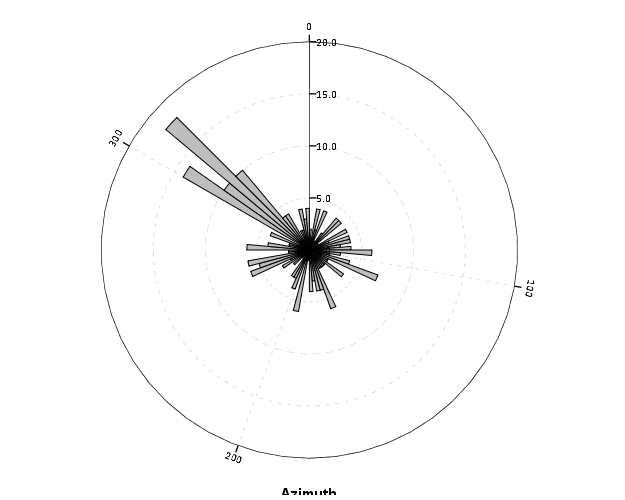So a simple solution is to offset the center of the plot, so the bars don’t start at the origin, but a prespecified distance away from the center of the circle. Below is the same chart previously with a slight offset. (I saw this idea originally in Wilkinson’s Grammar of Graphics.)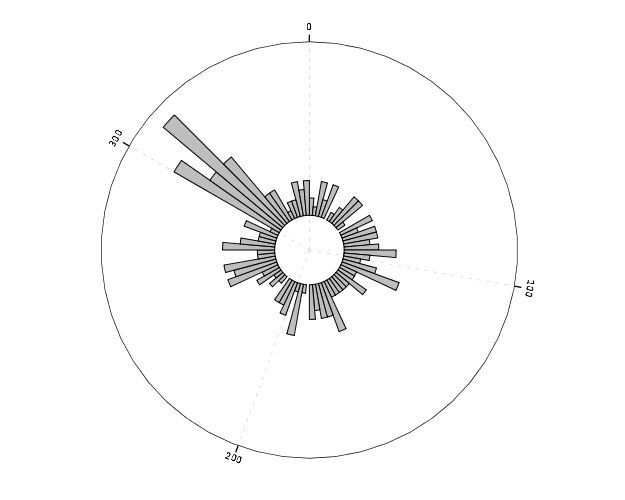And here is that technique extended to an example small multiple histogram from an earlier draft of the paper I previously mentioned.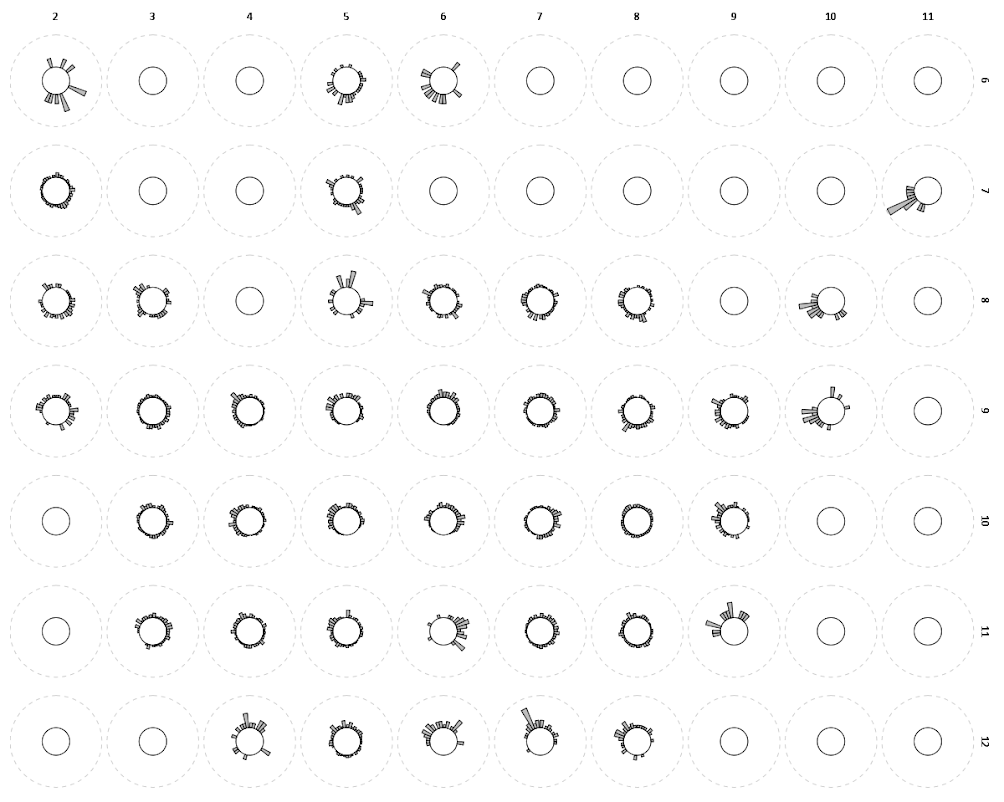Even with the offset, the problem of the shrinking area is even worse because of the many plots, and the outlying bars in one plot shrinks the rest of the distribution even more dramatically. So, even with the offsetting it is still quite difficult assess trends. Also note I don’t even bother to draw the radius guide lines. I noticed in some recent papers about analyzing circular data that they don’t draw bars for circular histograms, but use dots (and/or kernel density estimates). See examples in Brunsdon and Corcoran (2006), Ashby and Bowers (2013), and Russell and Levitin (1995). The below image is taken from Ashby and Bowers (2013) to demonstrate this.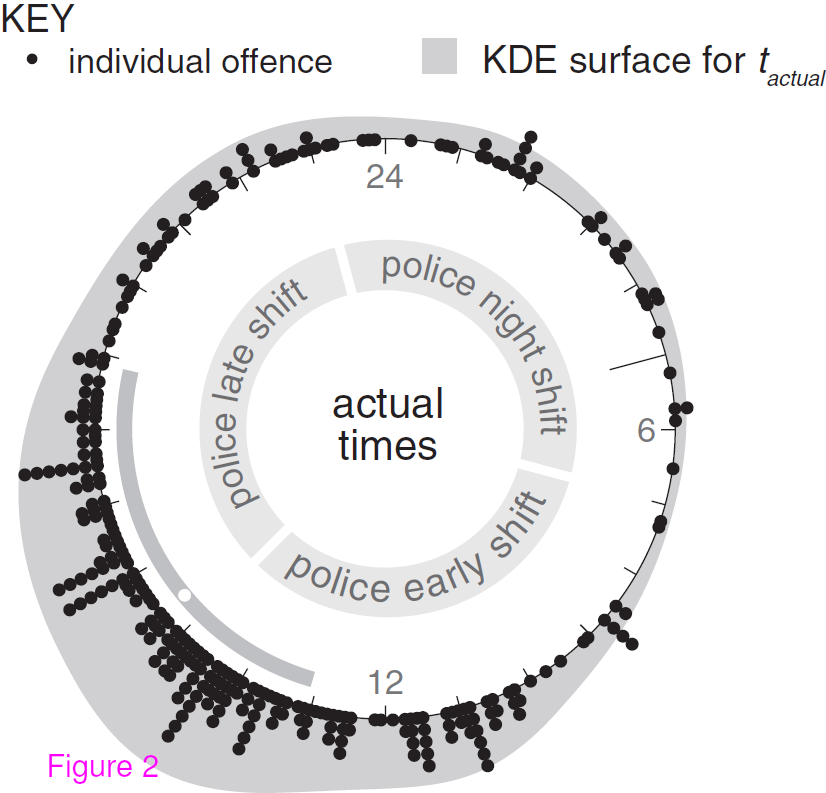The idea behind this is that, in polar coordinates, you need to measure the length of the bar, instead of distance from a common reference line. When you use dots, it is pretty trivial to just count the dots to see how far they stack up (so no axis guide is needed). This just replaces one problem for other ones, especially for larger sample sizes (in which you will need to discretize how many observations a point represents) but I don’t think it is any worse than bars at least in this situation (and can potentially be better for a smaller number of dots). One thing that does happen with points is that large stacks deviate from each other the further they grow towards the circumference of the polar coordinate system (the bars in histograms typically get wider). This just looks aesthetically bad, although the bars growing wider could be considered a disingenuous representation (e.g. Florence Nightingale’s coxcomb chart) (Brasseur, 2005; Friendly, 2008).

Unfortunately, SPSS’s routine to stack the dots in polar coordinates is off just slightly (I have full code linked at the end of the post to recreate some of the graphs in the post and display this behavior).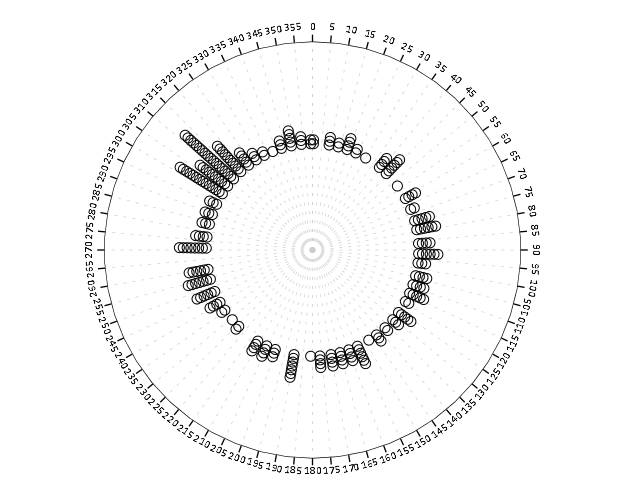With alittle data manipulation though you can basically roll your own (although this is fixed bins, unlike irregular ones chosen based on the data like in Wilkinson’s dot plots, e.g. `bin.dot` in GPL) (Wilkinson, 1999).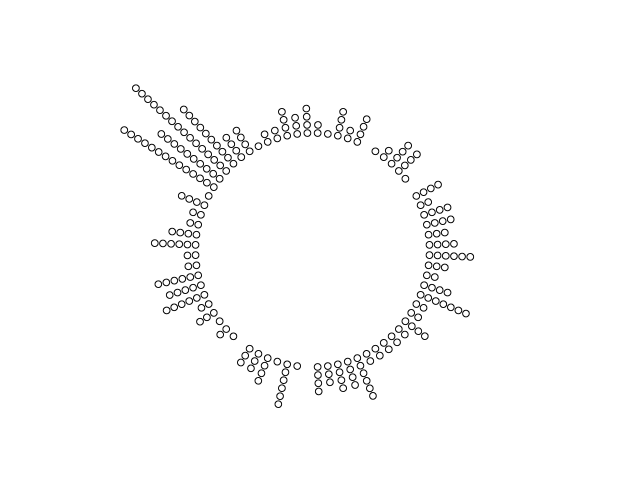And here is the same example small multiple histogram using the dots.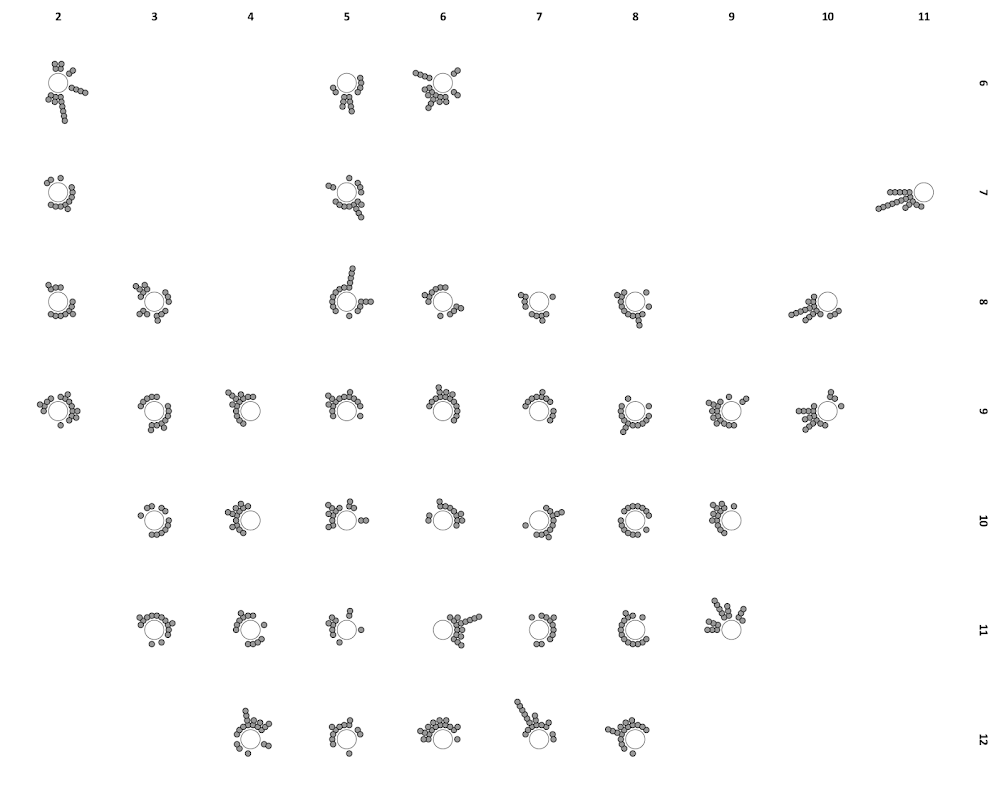Here I have posted the code to demonstrate some of the graphs here (and I have the full code for the Viz. JTC paper here). To make the circular dot plot I use the sequential case processing trick, and then show how to use `TRANS` statements in inline GPL to adjust the positioning of the dots and if you want the dots to represent multiple values.

# Some discussion on circular helio bar charts

The other day I saw a popular post on the mathematica site was to reconstruct helio plots. They are essentially bar charts of canonical correlation coefficients plotted in polar coordinates, and below is the most grandiose example of them I could find (Degani et al., 2006).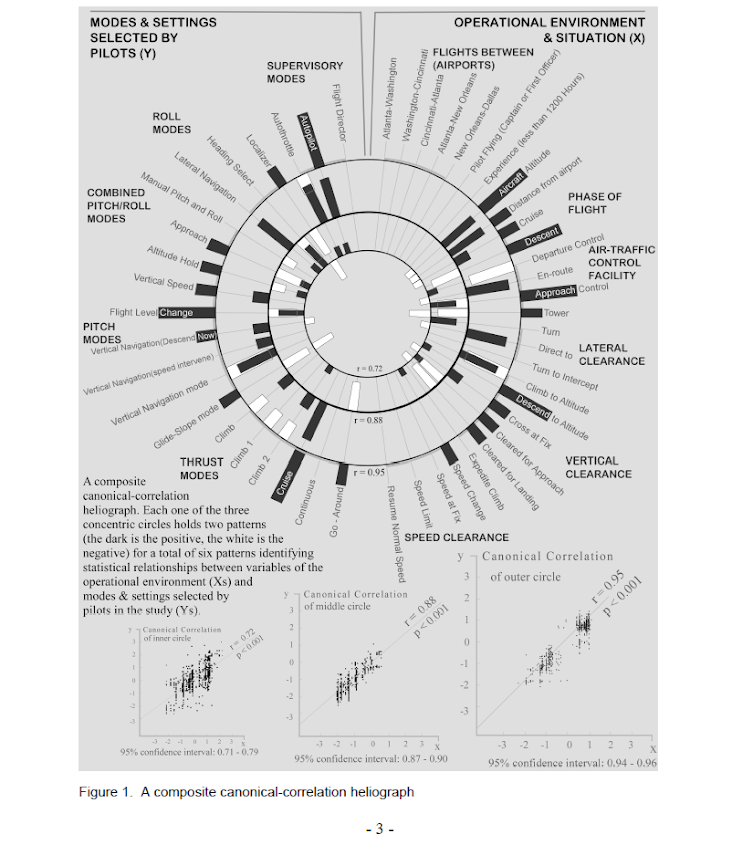That is a bit of a crazy example, but it is essentially several layers of bar charts in polar coordinates, with seperate rings displaying seperate correlation coefficients. Seeing there use in action struck me as odd, given typical perceptual problems known with using polar coordinates. Polar coordinates are popular for their space saving capabilities for network diagrams (see for example Circos) but there appears to be no redeeming quality of using polar coordinates for displaying the data in these circumstances that I can tell. The Degani paper gives the motivation for the polar coordinates because polar coordinates lack natural ordering that plots in cartesian coordinates imply. This strikes me as either unfounded or hypocritical, so I don’t really see why that is a reasonable motivation.

Polar coordinates have the negatives here that points going towards the center of the circle are compressed in smaller areas, and points going towards the edge of the circle are spread further apart. This creates a visual bias that does not portray actual data. I also presume length judgements in polar coordinates are more difficult. This having some bars protruding closer to one another and some diverging farther away I suspect cause more error judgements in false associations than do any ordering in bar charts in rectilinear coordinates. Also polar coordinates are very difficult to portray radial axis labels, so specific quantitative assements (e.g. this correlation is .5 and this correlation is .3) are difficult to make.

Below I will show an example taken from page 8 of Aboaja et al. (2011). Below is a screen shot of their helio plot, produced with the R package `yacca`.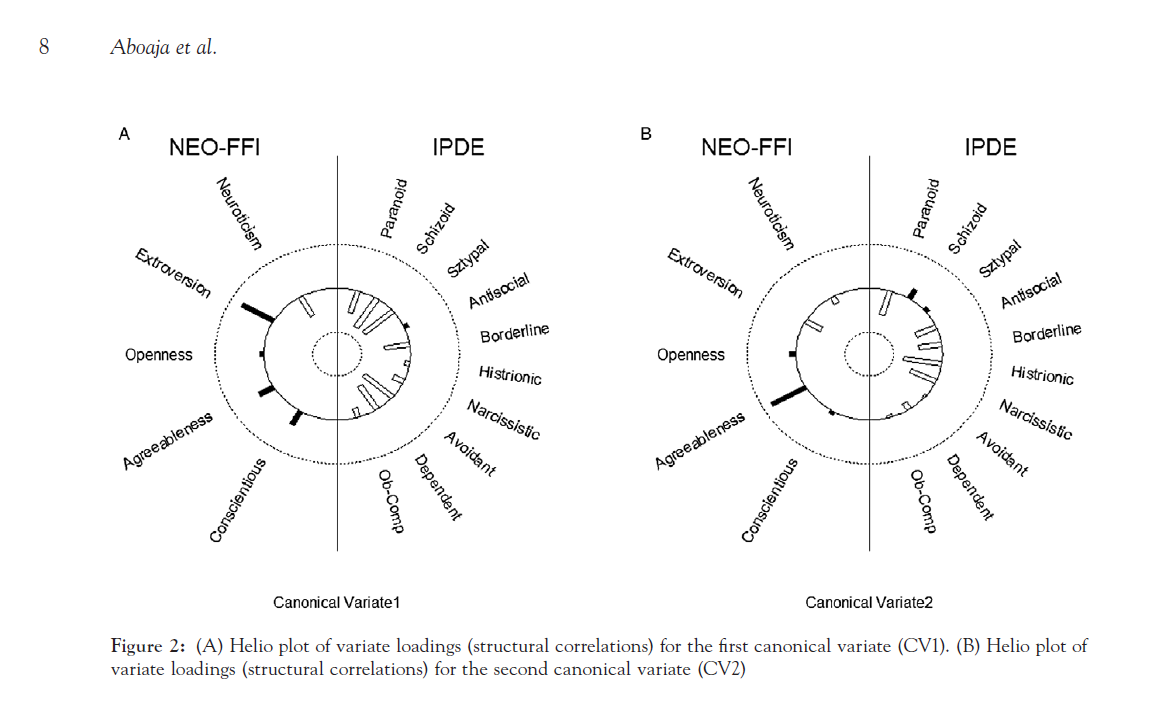So first, lets not go crazy and just see how a simple bar chart suffices to show the data. I use nesting here to differentiate between NEO-FFI and IPDE factors, but one could use other aesthetics like color or pattern to clearly distinguish between the two.

``````
data list free / type (F1.0) factors (F2.0) CV1 CV2.
begin data
1 1 -0.49 -0.17
1 2 0.73 -0.37
1 3 0.07 0.14
1 4 0.34 0.80
1 5 0.36 0.08
2 6 -0.53 -0.57
2 7 -0.78 0.25
2 8 -0.77 0.08
2 9 0.10 -0.45
2 10 -0.51 -0.48
2 11 -0.79 -0.48
2 12 -0.24 -0.56
2 13 -0.76 -0.04
2 14 -0.65 -0.16
2 15 -0.21 -0.05
end data.
value labels type
1 'NEO-FFI'
2 'IPDE'.
value labels factors
1 'Neuroticism'
2 'Extroversion'
3 'Openness'
4 'Agreeableness'
5 'Conscientiousness'
6 'Paranoid'
7 'Schizoid'
8 'Schizotypal'
9 'Antisocial'
10 'Borderline'
11 'Histrionic'
12 'Narcissistic'
13 'Avoidant'
14 'Dependent'
15 'Obsessive Compulsive'.
formats CV1 CV2 (F2.1).

*Bar Chart.
GGRAPH
/GRAPHDATASET NAME="graphdataset" VARIABLES=factors CV1 type
/GRAPHSPEC SOURCE=INLINE.
BEGIN GPL
SOURCE: s=userSource(id("graphdataset"))
DATA: factors=col(source(s), name("factors"), unit.category())
DATA: type=col(source(s), name("type"), unit.category())
DATA: CV1=col(source(s), name("CV1"))
GUIDE: axis(dim(1), opposite())
GUIDE: axis(dim(2), label("CV1"))
SCALE: cat(dim(1.1), include("1", "2", "3", "4", "5", "6", "7", "8", "9", "10", "11", "12", "13", "14", "15"))
SCALE: linear(dim(2), min(-1), max(1))
ELEMENT: interval(position(factors/type*CV1), shape.interior(shape.square))
END GPL.
``````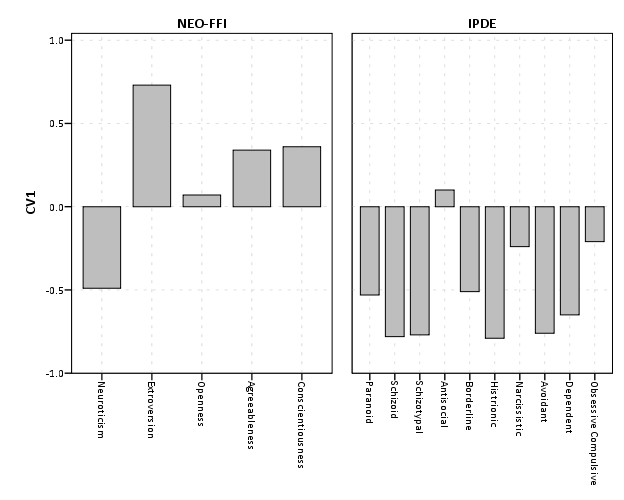This shows an example of using nesting for the faceting structure in SPSS. The default behavior for SPSS is that the NEO-FFI has fewer categories, so the bars are plotted wider (because the panels are set to be equally sized). Wilkinson’s Grammar has examples of setting the panels to be different sizes just in this situation, but I do not believe this is possible in SPSS. Because of this, I like to use point and edge elements to just symbolize lines, which makes the panels visually similar. Also I post-hoc added a guideline at the zero value and sorted the values of `CV1` descendingly within panels.

``````
*Because of different sizes - I like the line with dotted interval.
GGRAPH
/GRAPHDATASET NAME="graphdataset" VARIABLES=factors CV1 type
/GRAPHSPEC SOURCE=INLINE.
BEGIN GPL
SOURCE: s=userSource(id("graphdataset"))
DATA: factors=col(source(s), name("factors"), unit.category())
DATA: type=col(source(s), name("type"), unit.category())
DATA: CV1=col(source(s), name("CV1"))
TRANS: base=eval(0)
GUIDE: axis(dim(1), opposite())
GUIDE: axis(dim(2), label("CV1"))
SCALE: cat(dim(1.1), include("1", "2", "3", "4", "5", "6", "7", "8", "9", "10", "11", "12", "13", "14", "15"), sort.statistic(summary.max(CV1)), reverse())
SCALE: linear(dim(2), min(-1), max(1))
ELEMENT: edge(position(factors/type*(base+CV1)), shape.interior(shape.dash), color(color.grey))
ELEMENT: point(position(factors/type*CV1), shape.interior(shape.circle), color.interior(color.grey))
END GPL.
``````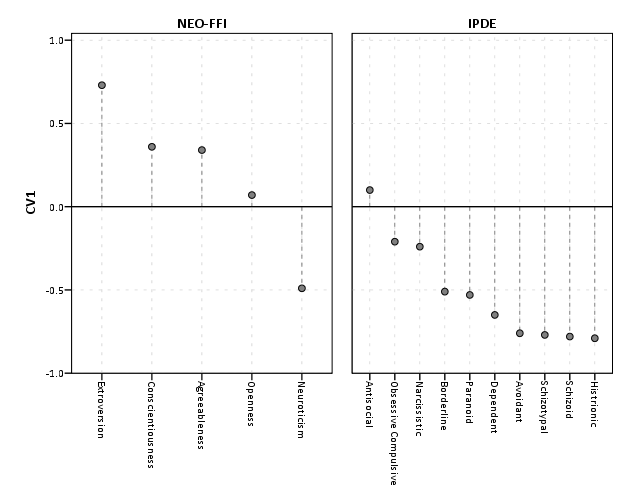If one wanted to show both variates within the same plot, one could either use panels (as did the original Aboaja article, just in polar coordinates) or one could superimpose those estimates on the same plot. An example of superimposing is given below. This superimposing also extends to more than two canonical variates, although the more points the more the graph gets so busy it is difficult to interpret and one might want to consider small multiples. Here I show superimposing `CV1` and `CV2` and sort by descending values of `CV2`.

``````
GGRAPH
/GRAPHDATASET NAME="graphdataset" VARIABLES=factors CV1 CV2 type
/GRAPHSPEC SOURCE=INLINE.
BEGIN GPL
SOURCE: s=userSource(id("graphdataset"))
DATA: factors=col(source(s), name("factors"), unit.category())
DATA: type=col(source(s), name("type"), unit.category())
DATA: CV1=col(source(s), name("CV1"))
DATA: CV2=col(source(s), name("CV2"))
TRANS: base=eval(0)
GUIDE: axis(dim(1), opposite())
GUIDE: axis(dim(2), label("CV"))
SCALE: cat(dim(1.1), include("1", "2", "3", "4", "5", "6", "7", "8", "9", "10", "11", "12", "13", "14", "15"), sort.statistic(summary.max(CV2)), reverse())
SCALE: linear(dim(2), min(-1), max(1))
ELEMENT: line(position(factors/type*base), color(color.black))
ELEMENT: point(position(factors/type*CV1), shape.interior("CV1"), color.interior("CV1"))
ELEMENT: point(position(factors/type*CV2), shape.interior("CV2"), color.interior("CV2"))
END GPL.
``````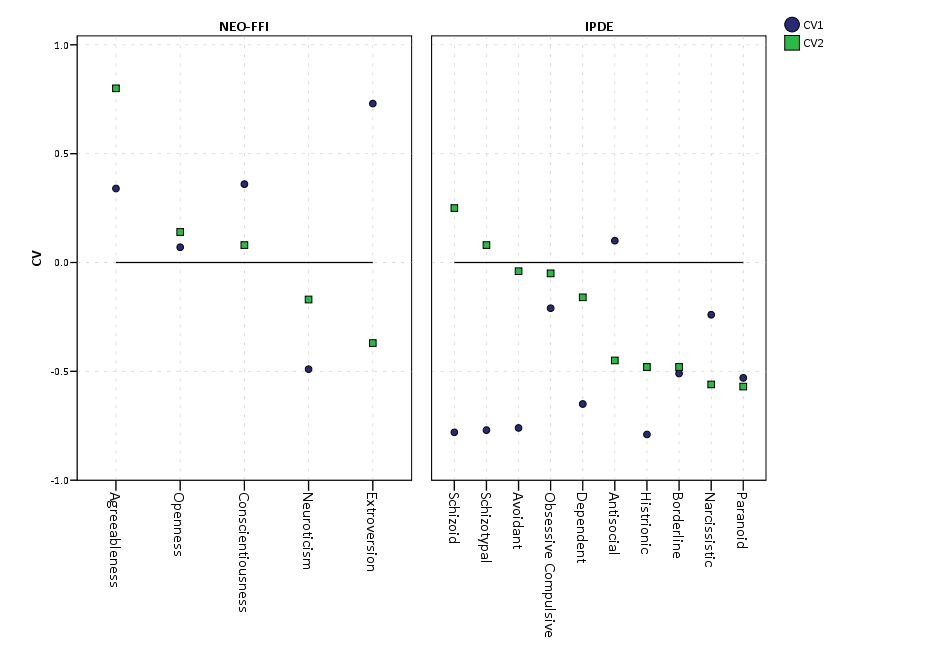Now, I know nothing of canonical correlation, but if one wanted to show the change from the first to second canonical covariate one could use the edge element with an arrow. One could also order the axis here, based on values of either the first or second canonical variate, or on the change between variates. Here I sort ascendingly by the absolute value in the change between variates.

``````
GGRAPH
/GRAPHDATASET NAME="graphdataset" VARIABLES=factors CV1 CV2 type
/GRAPHSPEC SOURCE=INLINE.
BEGIN GPL
SOURCE: s=userSource(id("graphdataset"))
DATA: factors=col(source(s), name("factors"), unit.category())
DATA: type=col(source(s), name("type"), unit.category())
DATA: CV1=col(source(s), name("CV1"))
DATA: CV2=col(source(s), name("CV2"))
TRANS: base=eval(0)
GUIDE: axis(dim(1), opposite())
GUIDE: axis(dim(2), label("CV"))
SCALE: cat(dim(1.1), include("1", "2", "3", "4", "5", "6", "7", "8", "9", "10", "11", "12", "13", "14", "15"), sort.statistic(summary.max(diff)))
SCALE: linear(dim(2), min(-1), max(1))
ELEMENT: line(position(factors/type*base), color(color.black))
ELEMENT: edge(position(factors/type*(CV1+CV2)), shape.interior(shape.arrow), color.interior(color.red))
ELEMENT: point(position(factors/type*CV1), shape.interior(shape.circle), color.interior(color.red))
END GPL.
``````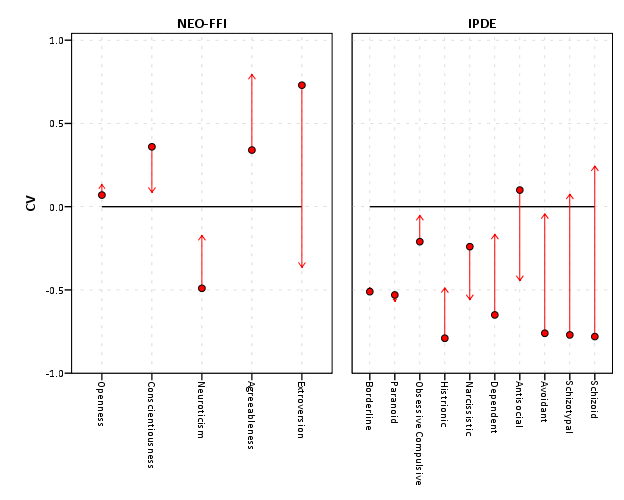I’ve posted some additional code at the end of the blog post to show the nuts and bolts of making a similar chart in polar coordinates, plus a few other potential variants like a clustered bar chart. I see little reason though to prefer them to more traditional bar charts in a rectilinear coordinate system.

``````
***********************************************************************************.
*Full code snippet.
data list free / type (F1.0) factors (F2.0) CV1 CV2.
begin data
1 1 -0.49 -0.17
1 2 0.73 -0.37
1 3 0.07 0.14
1 4 0.34 0.80
1 5 0.36 0.08
2 6 -0.53 -0.57
2 7 -0.78 0.25
2 8 -0.77 0.08
2 9 0.10 -0.45
2 10 -0.51 -0.48
2 11 -0.79 -0.48
2 12 -0.24 -0.56
2 13 -0.76 -0.04
2 14 -0.65 -0.16
2 15 -0.21 -0.05
end data.
value labels type
1 'NEO-FFI'
2 'IPDE'.
value labels factors
1 'Neuroticism'
2 'Extroversion'
3 'Openness'
4 'Agreeableness'
5 'Conscientiousness'
6 'Paranoid'
7 'Schizoid'
8 'Schizotypal'
9 'Antisocial'
10 'Borderline'
11 'Histrionic'
12 'Narcissistic'
13 'Avoidant'
14 'Dependent'
15 'Obsessive Compulsive'.
formats CV1 CV2 (F2.1).

*Bar Chart.
GGRAPH
/GRAPHDATASET NAME="graphdataset" VARIABLES=factors CV1 type
/GRAPHSPEC SOURCE=INLINE.
BEGIN GPL
SOURCE: s=userSource(id("graphdataset"))
DATA: factors=col(source(s), name("factors"), unit.category())
DATA: type=col(source(s), name("type"), unit.category())
DATA: CV1=col(source(s), name("CV1"))
GUIDE: axis(dim(1), opposite())
GUIDE: axis(dim(2), label("CV1"))
SCALE: cat(dim(1.1), include("1", "2", "3", "4", "5", "6", "7", "8", "9", "10", "11", "12", "13", "14", "15"))
SCALE: linear(dim(2), min(-1), max(1))
ELEMENT: interval(position(factors/type*CV1), shape.interior(shape.square))
END GPL.

*Because of different sizes - I like the line with dotted interval.
GGRAPH
/GRAPHDATASET NAME="graphdataset" VARIABLES=factors CV1 type
/GRAPHSPEC SOURCE=INLINE.
BEGIN GPL
SOURCE: s=userSource(id("graphdataset"))
DATA: factors=col(source(s), name("factors"), unit.category())
DATA: type=col(source(s), name("type"), unit.category())
DATA: CV1=col(source(s), name("CV1"))
TRANS: base=eval(0)
GUIDE: axis(dim(1), opposite())
GUIDE: axis(dim(2), label("CV1"))
SCALE: cat(dim(1.1), include("1", "2", "3", "4", "5", "6", "7", "8", "9", "10", "11", "12", "13", "14", "15"), sort.statistic(summary.max(CV1)), reverse())
SCALE: linear(dim(2), min(-1), max(1))
ELEMENT: edge(position(factors/type*(base+CV1)), shape.interior(shape.dash), color(color.grey))
ELEMENT: point(position(factors/type*CV1), shape.interior(shape.circle), color.interior(color.grey))
END GPL.

*Dot Plot Showing Both.
GGRAPH
/GRAPHDATASET NAME="graphdataset" VARIABLES=factors CV1 CV2 type
/GRAPHSPEC SOURCE=INLINE.
BEGIN GPL
SOURCE: s=userSource(id("graphdataset"))
DATA: factors=col(source(s), name("factors"), unit.category())
DATA: type=col(source(s), name("type"), unit.category())
DATA: CV1=col(source(s), name("CV1"))
DATA: CV2=col(source(s), name("CV2"))
TRANS: base=eval(0)
GUIDE: axis(dim(1), opposite())
GUIDE: axis(dim(2), label("CV"))
SCALE: cat(dim(1.1), include("1", "2", "3", "4", "5", "6", "7", "8", "9", "10", "11", "12", "13", "14", "15"), sort.statistic(summary.max(CV2)), reverse())
SCALE: linear(dim(2), min(-1), max(1))
ELEMENT: line(position(factors/type*base), color(color.black))
ELEMENT: point(position(factors/type*CV1), shape.interior("CV1"), color.interior("CV1"))
ELEMENT: point(position(factors/type*CV2), shape.interior("CV2"), color.interior("CV2"))
END GPL.

*Arrow going from CV1 to CV2.
GGRAPH
/GRAPHDATASET NAME="graphdataset" VARIABLES=factors CV1 CV2 type
/GRAPHSPEC SOURCE=INLINE.
BEGIN GPL
SOURCE: s=userSource(id("graphdataset"))
DATA: factors=col(source(s), name("factors"), unit.category())
DATA: type=col(source(s), name("type"), unit.category())
DATA: CV1=col(source(s), name("CV1"))
DATA: CV2=col(source(s), name("CV2"))
TRANS: diff=eval(abs(CV1 - CV2))
TRANS: base=eval(0)
GUIDE: axis(dim(1), opposite())
GUIDE: axis(dim(2), label("CV"))
SCALE: cat(dim(1.1), include("1", "2", "3", "4", "5", "6", "7", "8", "9", "10", "11", "12", "13", "14", "15"), sort.statistic(summary.max(diff)))
SCALE: linear(dim(2), min(-1), max(1))
ELEMENT: line(position(factors/type*base), color(color.black))
ELEMENT: edge(position(factors/type*(CV1+CV2)), shape.interior(shape.arrow), color.interior(color.red))
ELEMENT: point(position(factors/type*CV1), shape.interior(shape.circle), color.interior(color.red))
END GPL.

*If you must, polar coordinate helio like plot.
GGRAPH
/GRAPHDATASET NAME="graphdataset" VARIABLES=factors CV1 CV2 type
/GRAPHSPEC SOURCE=INLINE.
BEGIN GPL
SOURCE: s=userSource(id("graphdataset"))
DATA: factors=col(source(s), name("factors"), unit.category())
DATA: type=col(source(s), name("type"), unit.category())
DATA: CV1=col(source(s), name("CV1"))
DATA: CV2=col(source(s), name("CV2"))
TRANS: base=eval(0)
COORD: polar()
GUIDE: axis(dim(2), null())
SCALE: cat(dim(1), include("1", "2", "3", "4", "5", "6", "7", "8", "9", "10", "11", "12", "13", "14", "15"))
SCALE: linear(dim(2), min(-1), max(1))
ELEMENT: line(position(factors*base), color(color.black), closed())
ELEMENT: edge(position(factors*(base+CV1)), shape.interior(shape.dash), color.interior(type))
ELEMENT: point(position(factors*CV1), shape.interior(type), color.interior(type))
END GPL.

*Extras - not necesarrily recommended.

*Bars instead of lines in polar coordinates.
GGRAPH
/GRAPHDATASET NAME="graphdataset" VARIABLES=factors CV1 CV2 type
/GRAPHSPEC SOURCE=INLINE.
BEGIN GPL
SOURCE: s=userSource(id("graphdataset"))
DATA: factors=col(source(s), name("factors"), unit.category())
DATA: type=col(source(s), name("type"), unit.category())
DATA: CV1=col(source(s), name("CV1"))
DATA: CV2=col(source(s), name("CV2"))
TRANS: base=eval(0)
COORD: polar()
GUIDE: axis(dim(2), null())
SCALE: cat(dim(1), include("1", "2", "3", "4", "5", "6", "7", "8", "9", "10", "11", "12", "13", "14", "15"))
SCALE: linear(dim(2), min(-1), max(1))
ELEMENT: line(position(factors*base), color(color.black), closed())
ELEMENT: interval(position(factors*(base+CV1)), shape.interior(shape.square), color.interior(type))
END GPL.

*Clustering between CV1 and CV2? - need to reshape.
varstocases
/make CV from CV1 CV2
/index order.

value labels order
1 'CV1'
2 'CV2'.

*Clustered Bar.
GGRAPH
/GRAPHDATASET NAME="graphdataset" VARIABLES=factors type CV order
/GRAPHSPEC SOURCE=INLINE.
BEGIN GPL
SOURCE: s=userSource(id("graphdataset"))
DATA: factors=col(source(s), name("factors"), unit.category())
DATA: type=col(source(s), name("type"), unit.category())
DATA: CV=col(source(s), name("CV"))
DATA: order=col(source(s), name("order"), unit.category())
COORD: rect(dim(1,2))
GUIDE: axis(dim(3), label("factors"))
GUIDE: axis(dim(2), label("CV"))
GUIDE: legend(aesthetic(aesthetic.color.interior))
SCALE: cat(aesthetic(aesthetic.color.interior))
ELEMENT: interval.dodge(position(factors/type*CV)), color.interior(order),shape.interior(shape.square))
END GPL.
***********************************************************************************.
``````